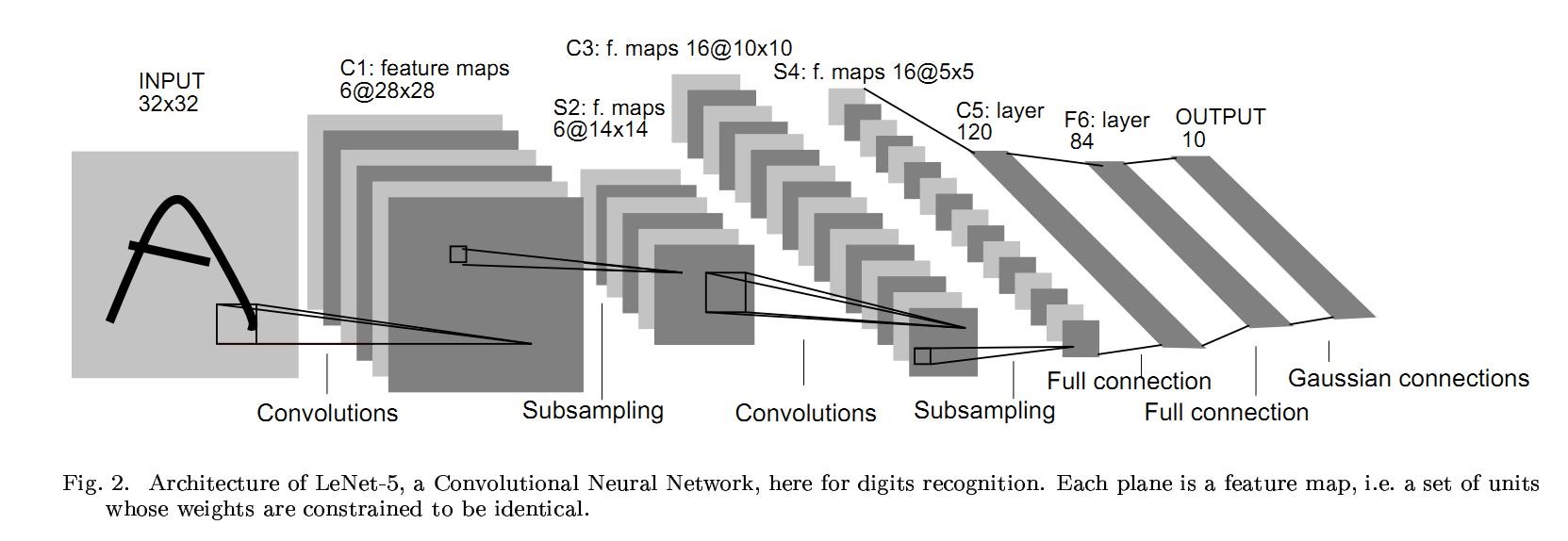# 0601-利用pytorch的nn工具箱实现LeNet网络

pytorch完整教程目录：https://www.cnblogs.com/nickchen121/p/14662511.html

# 一、引言# 二、定义网络

import torch as t
import torch.nn as nn
import torch.nn.functional as F
from torch.autograd import Variable as V

class Net(nn.Module):
def __init__(self):
super(Net, self).__init__()  # nn.Module 子类的函数必须在构造函数中执行父类的构造函数

# 卷积层
self.conv1 = nn.Conv2d(1, 6,
5)  # '1'表示输入图片为单通道，‘6’表示输出通道数，‘5’表示卷积核为 5*5
self.conv2 = nn.Conv2d(6, 16, 5)

# 仿射层/全连接层，y = Wx + b
self.fc1 = nn.Linear(16 * 5 * 5, 120)
self.fc2 = nn.Linear(120, 84)
self.fc3 = nn.Linear(84, 10)

def forward(self, x):
# 卷积-》激活-》池化
x = F.max_pool2d(F.relu(self.conv1(x)), (2, 2))
x = F.max_pool2d(F.relu(self.conv2(x)), 2)

# reshape，‘-1’表示自适应
x = x.view(x.size(), -1)
x = F.relu(self.fc1(x))
x = F.relu(self.fc2(x))
x = self.fc3(x)

return x

net = Net()
net

Net(
(conv1): Conv2d(1, 6, kernel_size=(5, 5), stride=(1, 1))
(conv2): Conv2d(6, 16, kernel_size=(5, 5), stride=(1, 1))
(fc1): Linear(in_features=400, out_features=120, bias=True)
(fc2): Linear(in_features=120, out_features=84, bias=True)
(fc3): Linear(in_features=84, out_features=10, bias=True)
)


1. 只要在 nn.Module 的子类中定义了 forward 函数，backward 函数就会自动实现
2. 网络的可学习参数通过 net.parameters() 返回，net.named_parameters 可同时返回可学习的参数和名称
3. 只有 Variable 才有自动求导功能，因此forward 函数的输入和输出都是 Variable，所以在输入时，需要把 Tensor 封装成 Variable
params = list(net.parameters())
len(params)

10

for name, parameters in net.named_parameters():
print(f'{name}: {parameters.size()}')

conv1.weight: torch.Size([6, 1, 5, 5])
conv1.bias: torch.Size()
conv2.weight: torch.Size([16, 6, 5, 5])
conv2.bias: torch.Size()
fc1.weight: torch.Size([120, 400])
fc1.bias: torch.Size()
fc2.weight: torch.Size([84, 120])
fc2.bias: torch.Size()
fc3.weight: torch.Size([10, 84])
fc3.bias: torch.Size()

input = V(t.randn(1, 1, 32, 32))  # 定义输入
out = net(input)
out.size()  # 输出的形状

torch.Size([1, 10])

net.zero_grad()  # 所有参数的梯度清零
out.backward(V(t.ones(1, 10)))  # 反向传播


# 三、损失函数

nn 实现了神经网络中大多数的损失函数，例如 nn.MSELoss 计算均方误差，nn.CrossEntropyLoss 计算交叉熵损失。

output = net(input)  # net(input)的输出的形状是(1,10)
target = V(t.arange(0, 10)).view(1, 10).float()
criterion = nn.MSELoss()
loss = criterion(output, target)
loss

tensor(28.5546, grad_fn=<MseLossBackward>)


input -> conv2d -> relu -> maxpool2d -> conv2d -> relu -> maxpool2d
-> view -> linear -> relu -> linear -> relu -> linear
-> MSELoss
-> loss


# 运行.backward，观察调用之前和调用之后的 grad
loss.backward()

反向传播之前conv1.bias 的梯度：tensor([0., 0., 0., 0., 0., 0.])



# 四、优化器

import torch.optim as optim

# 新建一个优化器，指定要调整的参数和学习率
optimizer = optim.SGD(net.parameters(), lr=0.01)

# 计算损失
output = net(input)
loss = criterion(output, target)

# 反向传播
loss.backward()

# 更新参数
optimizer.step()


# 六、Hub模块简介

import torch

model = torch.hub.load('pytorch/vision:v0.4.2', 'deeplabv3_resnet101', pretrained=True)  # 加载模型，第一次加载需要一点点时间
model.eval()  # 释放模型
`

# 七、总结

posted @ 2021-04-22 10:29  二十三岁的有德  阅读(226)  评论(0编辑  收藏